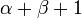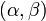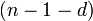# Regular graph

This article defines a property that can be evaluated to true/false for any undirected graph, and is invariant under graph isomorphism. Note that the term "undirected graph" as used here does not allow for loops or parallel edges, so there can be at most one edge between two distinct vertices, the edge is completely described by the vertices it joins, and there can be no edge from a vertex to itself.
View other such properties

## Definition

### For finite degrees

Supposeis a nonnegative integer. An undirected graph is termed-regular or degree-regular if it satisfies the following equivalent definitions:

1. The degrees of all vertices of the graph are equal to. Note thatmust be strictly less than the total number of vertices in the graph.
2. The adjacency matrix of the graph istimes a doubly stochastic matrix.

Note that if the graph is a finite graph, then we need only concern ourselves with the definition above for finite degrees.

### For arbitrary degrees

Supposeis a graph andare cardinals such thatequals the number of vertices in. Then,is regular for the pairif the degree of every vertex inisand the degree of every vertex in the complement ofis.

Note that ifis finite, this reduces to the definition in the finite case.

## Particular cases

Condition on number of vertices Condition on degree What can we say about the graph? Number of isomorphism classes possible after fixing number of vertices and degree
any 0 empty graph (graph with no edges) 1
2 1 2-clique (two vertices with an edge joining them) 1
even or infinite 1 matching graph: a disjoint union of 2-cliques 1
odd finite 1 no possibility 0
finite number2 union of pairwise disjoint cyclic graphs with cycle lengths of size at least three number of unordered integer partitions where all parts are at least 3
infinite 2 union of pairwise disjoint cyclic graphs and chains extending infinitely in both directions infinite
finite numbercomplete graph (i.e., clique) onvertices) 1
finite numbercomplement of a matching graph 1

## Relation with other properties

### Stronger properties

Property Meaning Proof of implication Proof of strictness (reverse implication failure) Intermediate notions
vertex-transitive graph automorphism group is transitive on vertex set vertex-transitive implies regular regular not implies vertex-transitive, regular connected not implies vertex-transitive Template:Intermediate notion short
complementation-closed graph property Yes complement of regular graph is regular The complement of a regular graph is regular. In the finite case, the complement of a-regular-vertex graph is-regular.